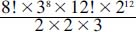NextPrevious

# What are the number of possible positions for a Rubik’s Cube?

Rubik’s Cube was invented in the 1970s by the Hungarian architect, inventor, and mathematician Ernö Rubik (1944-), who also invented a number of other puzzles, including Rubik’s Clock. The cube measures 3 by 3 by 3, with a total of 26 subcubes on the outside. All the subcubes are hinged, making them easy to turn (by a quarter turn in either direction) in any of the planes on the cube. Initially, each of the six sides are painted a certain color; the object is to move the cube planes in a random way, then return the cube so that each side has a single color again.

What are the possible number of positions of a Rubik’s cube? Mathematicians need to use factorials (symbolized with the ! sign; for more information about factorials, see “Algebra”) in order to find out the many iterations, as seen in the following equation:The number of positions turns out to be 43,252,003,274,489,856,000, or more than 43 quintillion turns.

Close

This is a web preview of the "The Handy Math Answer Book" app. Many features only work on your mobile device. If you like what you see, we hope you will consider buying. Get the App